One thing to note is that the code changes you have to make between questions often are minimal. Yet we go from running a simple binomial model to running a pretty advanced linear model.

## Question I

Not much to do here, other than to run it. Here is the graph you would see if everything is working properly.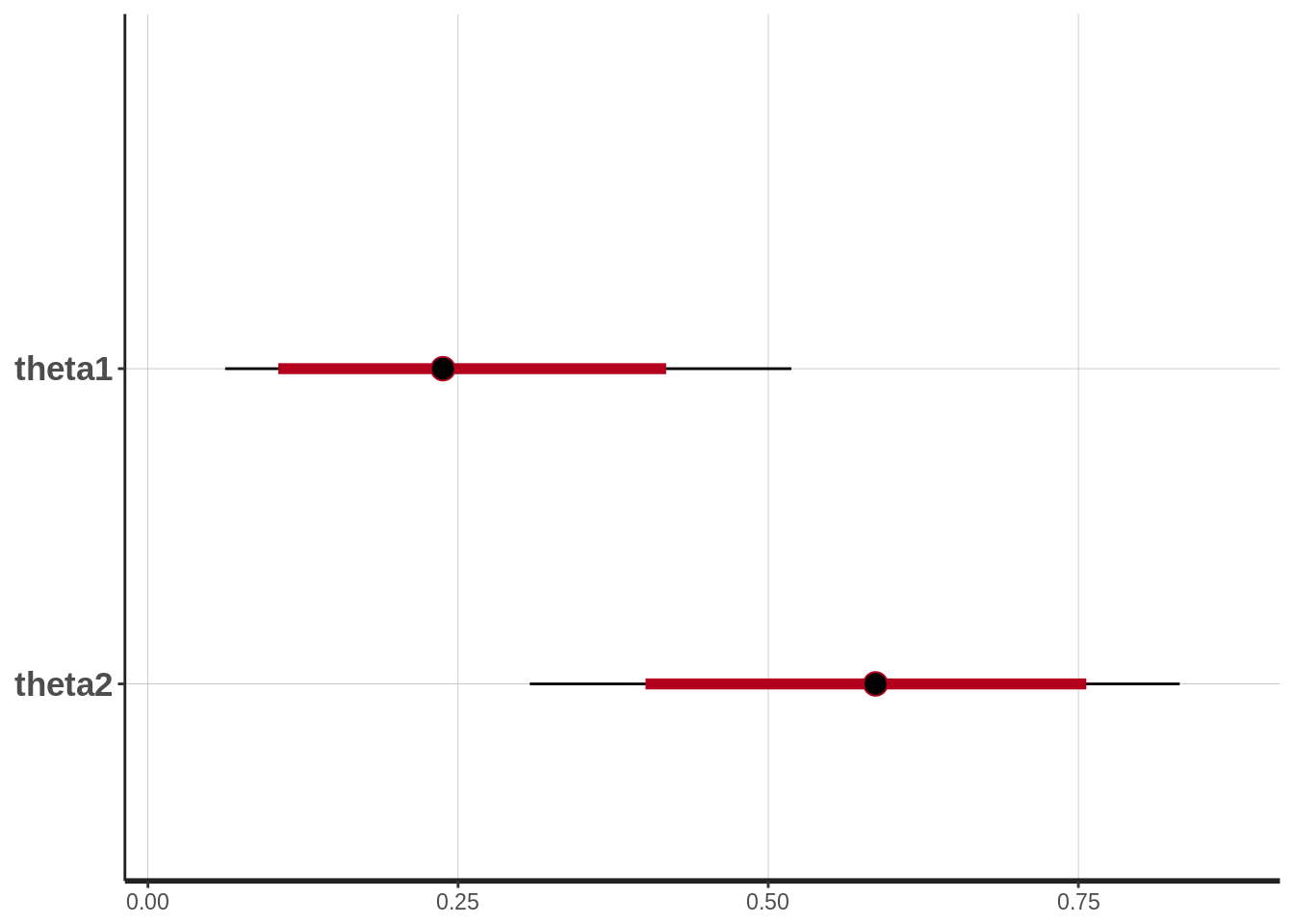## Question 2

``````s <- as.data.frame(stan_samples)
mean(abs(s\$theta2 - s\$theta1) < 0.2)``````
``##  0.2355``

## Question 3

``````cowA <- c(0, 1, 0, 0, 0, 0, 1, 0, 0, 0)
cowB <- c(0, 0, 1, 1, 1, 0, 1, 1, 1, 0)

# Using the same model as in Question 1, just using the new data.

data_list <- list(y1 = cowA, y2 = cowB, n1 = length(cowA), n2 = length(cowB))

# Compiling and producing posterior samples from the model.
stan_samples <- stan(model_code = model_string, data = data_list)``````
``````# Plotting and summarizing the posterior distribution
stan_samples``````
``````## Inference for Stan model: dc298a19c9e8503d8b6766e7e6e386e0.
## 4 chains, each with iter=2000; warmup=1000; thin=1;
## post-warmup draws per chain=1000, total post-warmup draws=4000.
##
##          mean se_mean   sd   2.5%    25%    50%    75%  97.5% n_eff Rhat
## theta1   0.25    0.00 0.12   0.06   0.16   0.23   0.33   0.53  3217    1
## theta2   0.58    0.00 0.14   0.30   0.49   0.59   0.69   0.83  3535    1
## lp__   -15.99    0.03 1.09 -18.97 -16.39 -15.64 -15.21 -14.94  1729    1
##
## Samples were drawn using NUTS(diag_e) at Sat Jan 14 23:34:43 2017.
## For each parameter, n_eff is a crude measure of effective sample size,
## and Rhat is the potential scale reduction factor on split chains (at
## convergence, Rhat=1).``````
``plot(stan_samples)``
``## ci_level: 0.8 (80% intervals)``
``## outer_level: 0.95 (95% intervals)``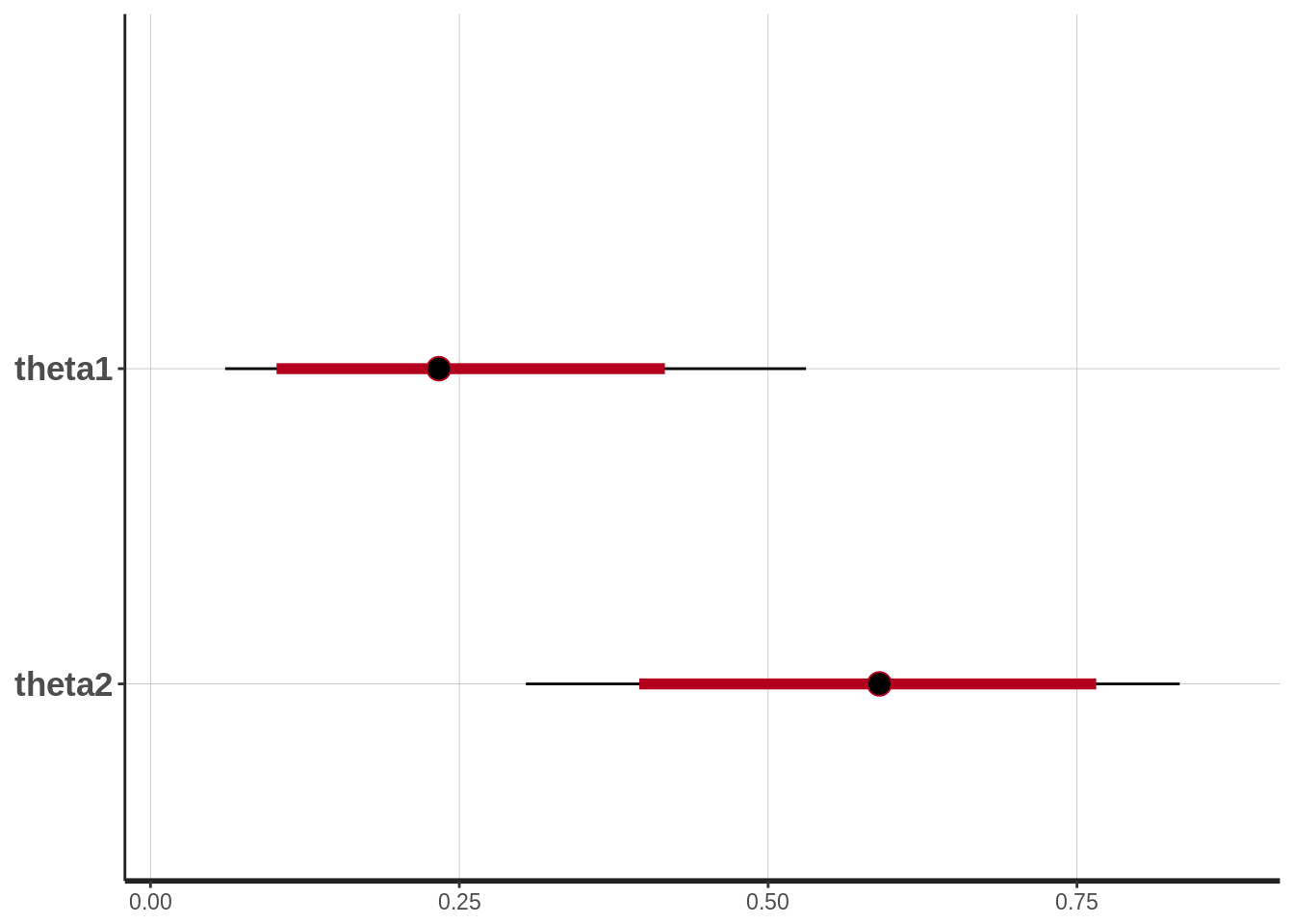Calculate the probability that medicine A is better than medicine B.

``````s <- as.data.frame(stan_samples)
mean(s\$theta1 > s\$theta2)``````
``##  0.04325``
``mean(s[,"theta1"] > s[,"theta2"])``
``##  0.04325``

So should probably go with medicine B then…

## Question 4

``````# The Stan model as a string.
model_string <- "
data {
int n1;
int n2;
vector[n1] y1;
vector[n2] y2;
}

parameters {
real mu1;
real mu2;
real<lower=0> sigma1;
real<lower=0> sigma2;
}

model {
mu1 ~ uniform(0, 2000);
mu2 ~ uniform(0, 2000);
sigma1 ~ uniform(0, 1000);
sigma2 ~ uniform(0, 1000);
y1 ~ normal(mu1, sigma1);
y2 ~ normal(mu2, sigma2);
}

generated quantities {
}
"

diet_milk <- c(651, 679, 374, 601, 401, 609, 767, 709, 704, 679)
normal_milk <- c(798, 1139, 529, 609, 553, 743, 151, 544, 488, 555, 257, 692, 678, 675, 538)
data_list <- list(y1 = diet_milk, y2 = normal_milk,
n1 = length(diet_milk), n2 = length(normal_milk))

# Compiling and producing posterior samples from the model.
stan_samples <- stan(model_code = model_string, data = data_list)``````
``````## Warning: There were 11 divergent transitions after warmup. Increasing adapt_delta above 0.8 may help. See
## https://mc-stan.org/misc/warnings.html#divergent-transitions-after-warmup``````
``## Warning: Examine the pairs() plot to diagnose sampling problems``
``````# Plotting and summarizing the posterior distribution
stan_samples``````
``````## Inference for Stan model: ce9fc71c270eed0bb9482a8456fb506d.
## 4 chains, each with iter=2000; warmup=1000; thin=1;
## post-warmup draws per chain=1000, total post-warmup draws=4000.
##
##           mean se_mean    sd    2.5%     25%     50%     75%   97.5% n_eff
## mu1     617.89    0.96 50.71  517.96  586.71  616.85  648.81  721.91  2767
## mu2     596.62    1.17 66.70  463.94  554.65  597.19  638.89  728.69  3234
## sigma1  153.61    0.95 47.10   93.27  121.91  143.81  173.81  273.19  2458
## sigma2  249.44    0.95 53.75  170.13  211.69  241.01  278.38  376.50  3211
## lp__   -133.48    0.04  1.58 -137.52 -134.27 -133.13 -132.31 -131.51  1548
##        Rhat
## mu1       1
## mu2       1
## sigma1    1
## sigma2    1
## lp__      1
##
## Samples were drawn using NUTS(diag_e) at Sat Jan 14 23:35:23 2017.
## For each parameter, n_eff is a crude measure of effective sample size,
## and Rhat is the potential scale reduction factor on split chains (at
## convergence, Rhat=1).``````
``plot(stan_samples)``
``## ci_level: 0.8 (80% intervals)``
``## outer_level: 0.95 (95% intervals)``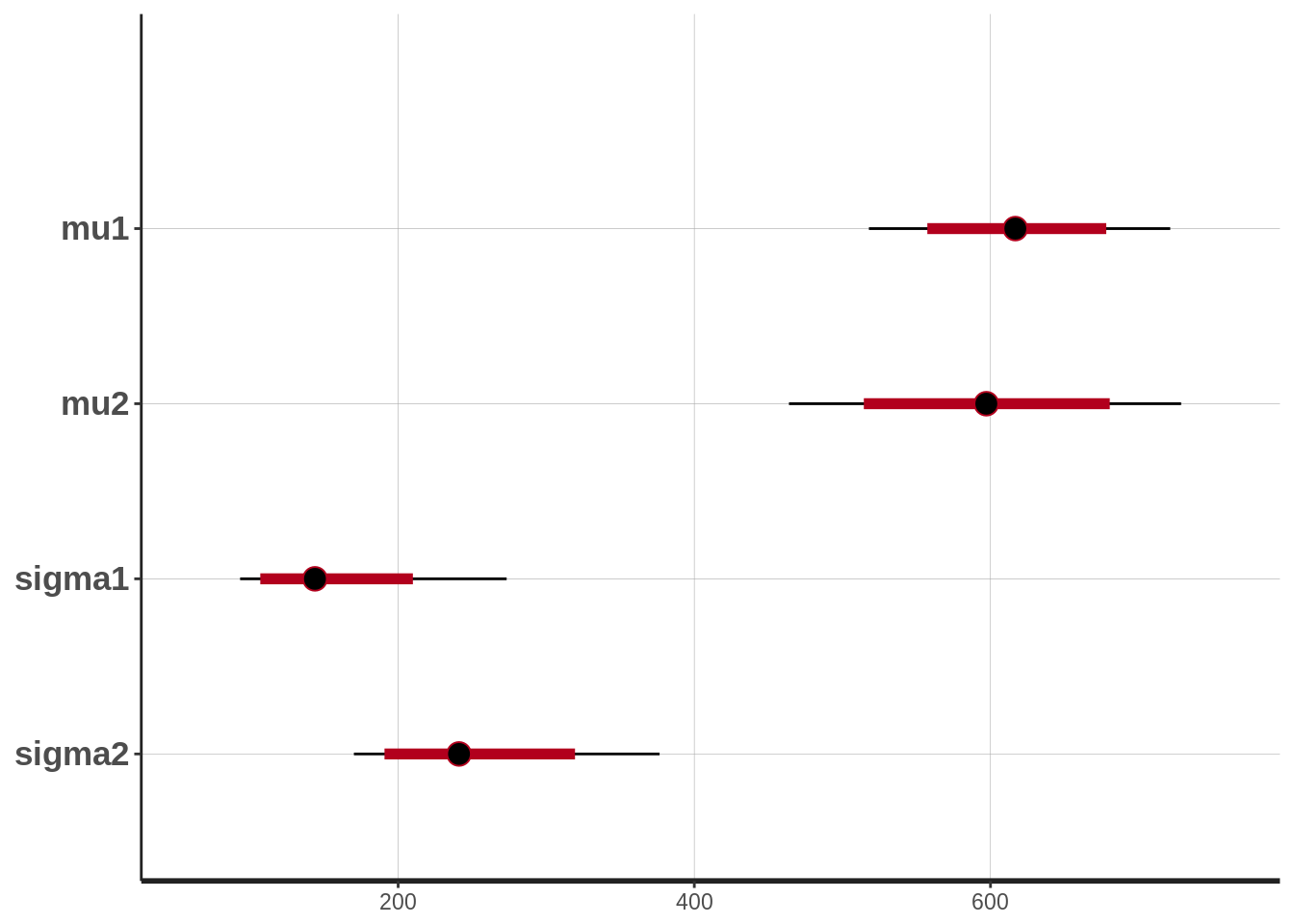Is it likely that the diet is going to make the cows produce more milk on average?

``````s <- as.data.frame(stan_samples)
mu_diff <- s\$mu2 - s\$mu1
hist(mu_diff)``````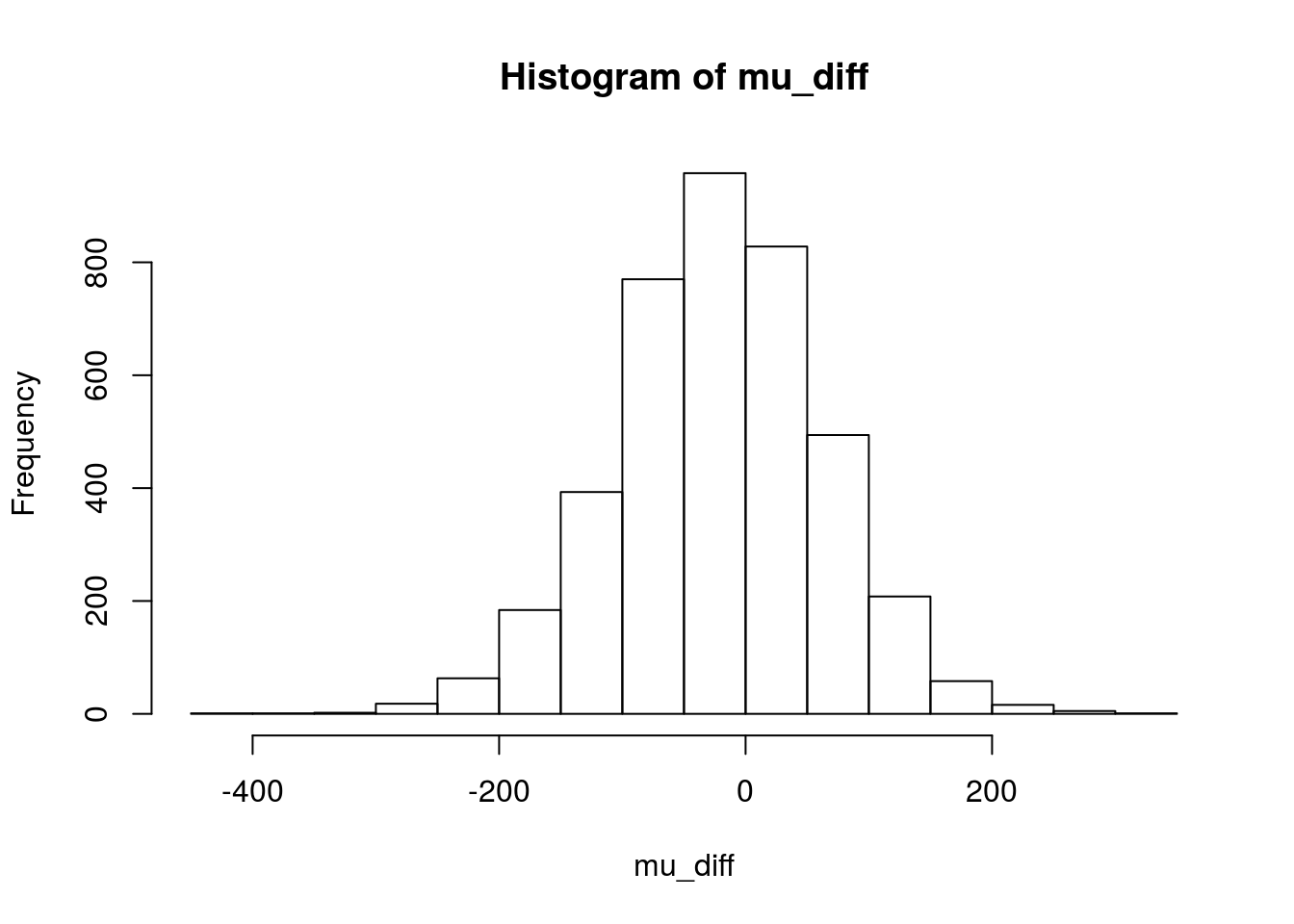``mean(mu_diff > 0)``
``##  0.4025``
``mean(mu_diff < 0)``
``##  0.5975``

It is almost as likely that the diet is better as that the diet is worse. So this experiment does not really support that the diet will result in the cows producing more milk .

## Question 5

``````# The Stan model as a string.
model_string <- "
data {
int n1;
int n2;
vector[n1] y1;
vector[n2] y2;
}

parameters {
real mu1;
real mu2;
real<lower=0> sigma1;
real<lower=0> sigma2;
}

model {
mu1 ~ uniform(0, 2000);
mu2 ~ uniform(0, 2000);
sigma1 ~ uniform(0, 1000);
sigma2 ~ uniform(0, 1000);
y1 ~ student_t(3, mu1, sigma1);
y2 ~ student_t(3, mu2, sigma2);
}

generated quantities {
}
"

diet_milk <- c(651, 679, 374, 601, 4000, 401, 609, 767, 3890, 704, 679)
normal_milk <- c(798, 1139, 529, 609, 553, 743, 3,151, 544, 488, 15, 257, 692, 678, 675, 538)
data_list <- list(y1 = diet_milk, y2 = normal_milk,
n1 = length(diet_milk), n2 = length(normal_milk))

# Compiling and producing posterior samples from the model.
stan_samples <- stan(model_code = model_string, data = data_list)``````
``````## Warning: There were 652 divergent transitions after warmup. Increasing adapt_delta above 0.8 may help. See
## https://mc-stan.org/misc/warnings.html#divergent-transitions-after-warmup``````
``## Warning: Examine the pairs() plot to diagnose sampling problems``
``````# Plotting and summarizing the posterior distribution
stan_samples``````
``````## Inference for Stan model: 5494eeeda3320aee4c85504377e391da.
## 4 chains, each with iter=2000; warmup=1000; thin=1;
## post-warmup draws per chain=1000, total post-warmup draws=4000.
##
##           mean se_mean     sd    2.5%     25%     50%     75%   97.5%
## mu1     655.78    3.53 138.64  389.64  573.29  645.48  728.69  968.33
## mu2     555.75    1.53  71.86  410.16  511.69  558.51  602.64  693.14
## sigma1  422.11    5.43 206.23  144.28  261.40  375.41  543.98  931.64
## sigma2  245.35    1.40  68.18  137.11  195.69  237.06  285.86  393.77
## lp__   -167.13    0.04   1.38 -170.64 -167.81 -166.85 -166.12 -165.37
##        n_eff Rhat
## mu1     1540    1
## mu2     2197    1
## sigma1  1445    1
## sigma2  2375    1
## lp__    1427    1
##
## Samples were drawn using NUTS(diag_e) at Sat Jan 14 23:36:07 2017.
## For each parameter, n_eff is a crude measure of effective sample size,
## and Rhat is the potential scale reduction factor on split chains (at
## convergence, Rhat=1).``````
``plot(stan_samples)``
``## ci_level: 0.8 (80% intervals)``
``## outer_level: 0.95 (95% intervals)``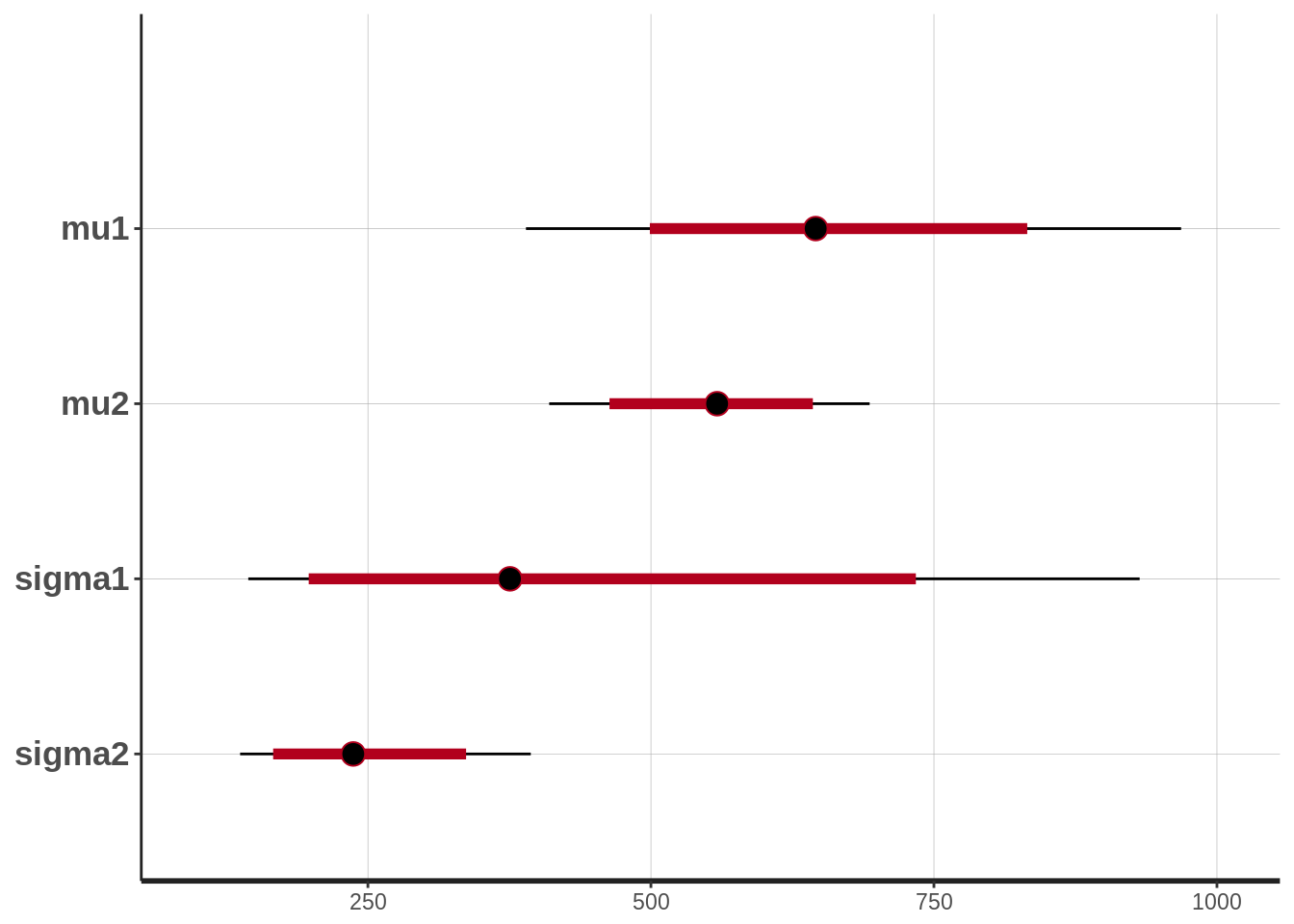Is it likely that diet is going to make the cows produce more milk on average?

``````s <- as.data.frame(stan_samples)
mu_diff <- s\$mu2 - s\$mu1
hist(mu_diff)``````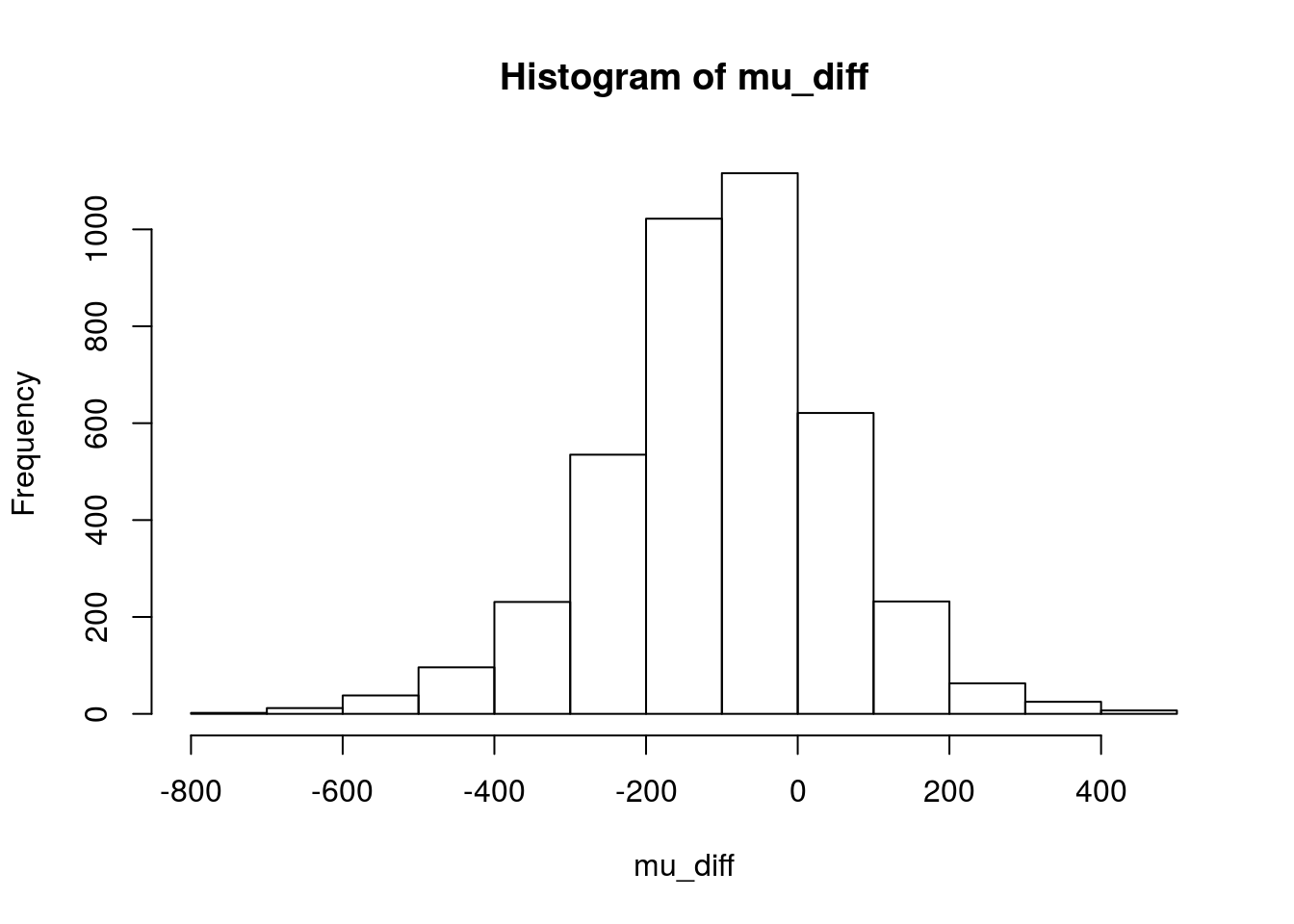``mean(mu_diff > 0) ``
``##  0.237``
``mean(mu_diff < 0)``
``##  0.763``

Again there is no strong evidence that the diet is any good (but compare with the result, would you have used the original Normal model!).

## Question 6

``````# The Stan model as a string.
model_string <- "
data {
int n1;
int n2;
int y1[n1];
int y2[n2];
}

parameters {
real<lower=0> lambda1;
real<lower=0> lambda2;
}

model {
lambda1 ~ uniform(0, 100);
lambda2 ~ uniform(0, 100);
y1 ~ poisson(lambda1);
y2 ~ poisson(lambda2);
}

generated quantities {
}
"

diet_eggs <- c(6, 4, 2, 3, 4, 3, 0, 4, 0, 6, 3)
normal_eggs <- c(4, 2, 1, 1, 2, 1, 2, 1, 3, 2, 1)
data_list <- list(y1 = diet_eggs, y2 = normal_eggs,
n1 = length(diet_eggs), n2 = length(normal_eggs))

# Compiling and producing posterior samples from the model.
stan_samples <- stan(model_code = model_string, data = data_list)``````
``````# Plotting and summarizing the posterior distribution
stan_samples``````
``````## Inference for Stan model: 03c92e31683dae13696b3e76eed7a9ec.
## 4 chains, each with iter=2000; warmup=1000; thin=1;
## post-warmup draws per chain=1000, total post-warmup draws=4000.
##
##          mean se_mean   sd  2.5%   25%   50%   75% 97.5% n_eff Rhat
## lambda1  3.26    0.01 0.54  2.29  2.87  3.23  3.61  4.36  3626    1
## lambda2  1.92    0.01 0.41  1.19  1.63  1.89  2.17  2.81  3655    1
## lp__    -1.72    0.02 0.99 -4.49 -2.09 -1.41 -1.02 -0.76  1820    1
##
## Samples were drawn using NUTS(diag_e) at Sat Jan 14 23:36:50 2017.
## For each parameter, n_eff is a crude measure of effective sample size,
## and Rhat is the potential scale reduction factor on split chains (at
## convergence, Rhat=1).``````
``plot(stan_samples)``
``## ci_level: 0.8 (80% intervals)``
``## outer_level: 0.95 (95% intervals)``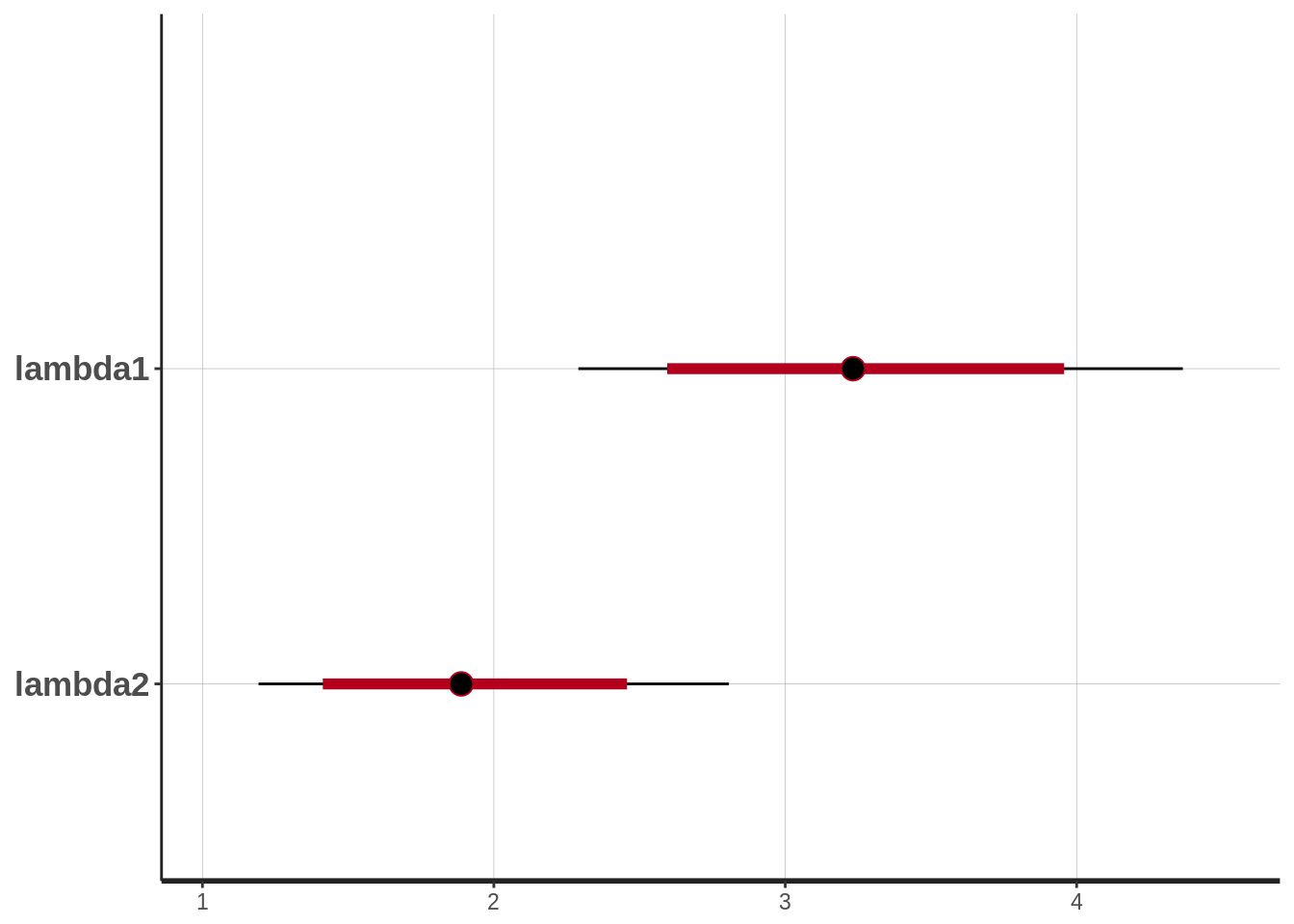Is it likely that diet going to make the chickens produce more eggs on average?

``````s <- as.data.frame(stan_samples)
lambda_diff <- s\$lambda1 - s\$lambda2
hist(lambda_diff)``````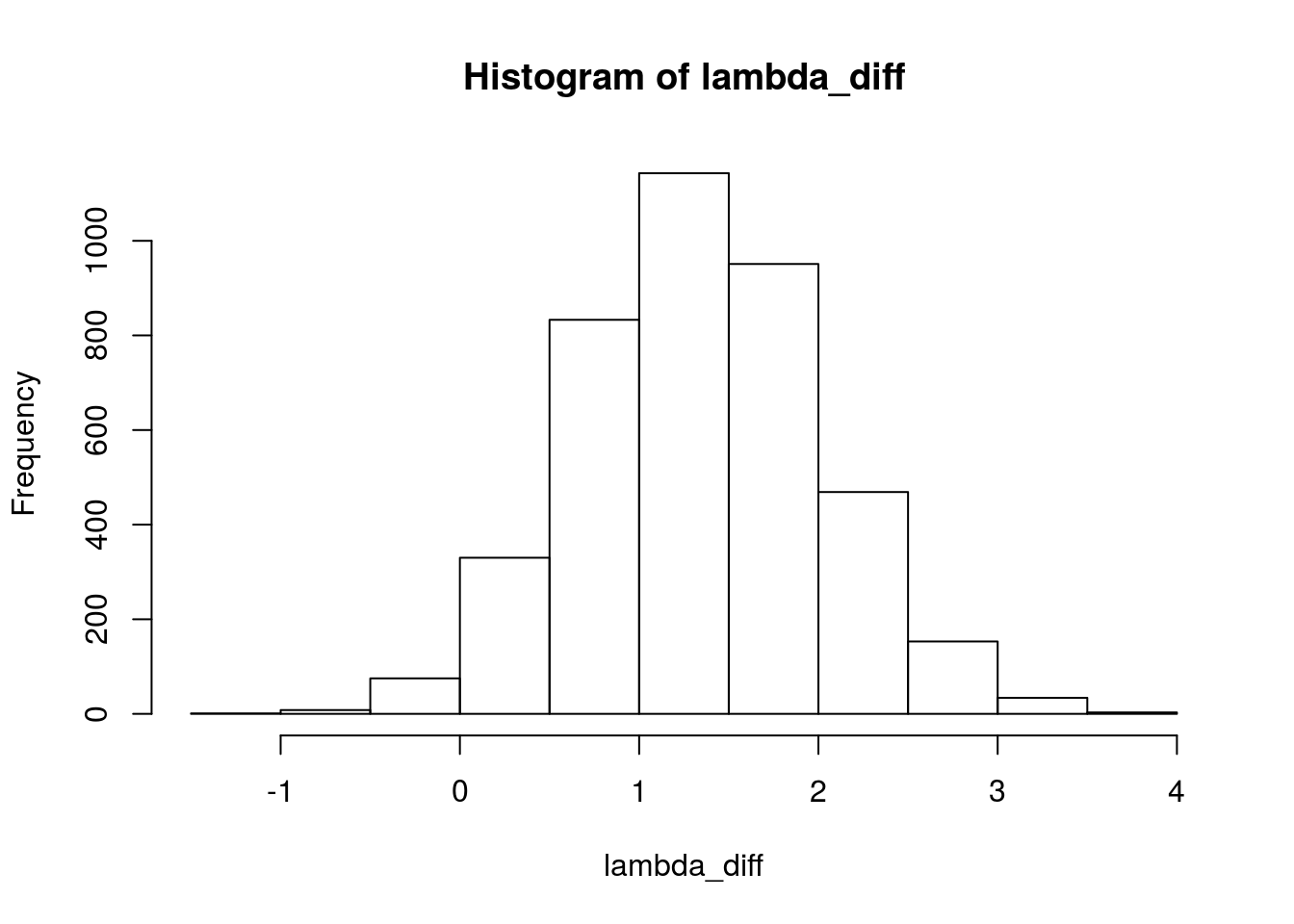``mean(lambda_diff > 0)``
``##  0.979``

There is pretty good evidence that the diet is effective and that chickens on the diet produce more eggs on average (that is, lambda1 seems higher than lambda2). Looking at `lambda_diff` a “best guess” is that the diet results in around 1-2 more eggs on average.

## Question 7

This implements the same model as in question 4, but using smarter indexing so that the code is not as redundant and so that it works with the format of the data in the data.frame `d` .

``````# The Stan model as a string.
model_string <- "
data {
int n;
int n_groups;
int x[n];
vector[n] y;
}

parameters {
vector[n_groups] mu;
vector<lower=0>[n_groups] sigma;
}

model {
mu ~ uniform(0, 2000);
sigma ~ uniform(0, 1000);
y ~ normal(mu[x], sigma[x]);
}

generated quantities {
}
"

d <- data.frame(
milk = c(651, 679, 374, 601, 401, 609, 767, 709, 704, 679, 798, 1139,
529, 609, 553, 743, 151, 544, 488, 555, 257, 692, 678, 675, 538),
group = c(1, 1, 1, 1, 1, 1, 1, 1, 1, 1, 2, 2,
2, 2, 2, 2, 2, 2, 2, 2, 2, 2, 2, 2, 2))
data_list <- list(y = d\$milk, x = d\$group, n = length(d\$milk),
n_groups = max(d\$group))

# Compiling and producing posterior samples from the model.
stan_samples <- stan(model_code = model_string, data = data_list)``````
``````## Warning: There were 6 divergent transitions after warmup. Increasing adapt_delta above 0.8 may help. See
## https://mc-stan.org/misc/warnings.html#divergent-transitions-after-warmup``````
``## Warning: Examine the pairs() plot to diagnose sampling problems``
``````# Plotting and summarizing the posterior distribution
stan_samples``````
``````## Inference for Stan model: 68927fed8cab7398aeed267d67b0a586.
## 4 chains, each with iter=2000; warmup=1000; thin=1;
## post-warmup draws per chain=1000, total post-warmup draws=4000.
##
##             mean se_mean    sd    2.5%     25%     50%     75%   97.5%
## mu     619.09    0.90 49.59  521.19  588.74  619.46  649.66  716.48
## mu     597.70    1.25 67.11  466.32  556.24  597.73  638.87  734.60
## sigma  152.78    0.89 44.91   93.89  121.71  143.90  173.62  263.09
## sigma  249.36    1.06 54.13  170.64  212.63  240.32  277.17  376.44
## lp__     -133.45    0.04  1.63 -137.52 -134.15 -133.04 -132.32 -131.52
##          n_eff Rhat
## mu     3043    1
## mu     2895    1
## sigma  2571    1
## sigma  2614    1
## lp__      1621    1
##
## Samples were drawn using NUTS(diag_e) at Sat Jan 14 23:37:33 2017.
## For each parameter, n_eff is a crude measure of effective sample size,
## and Rhat is the potential scale reduction factor on split chains (at
## convergence, Rhat=1).``````
``plot(stan_samples)``
``## ci_level: 0.8 (80% intervals)``
``## outer_level: 0.95 (95% intervals)``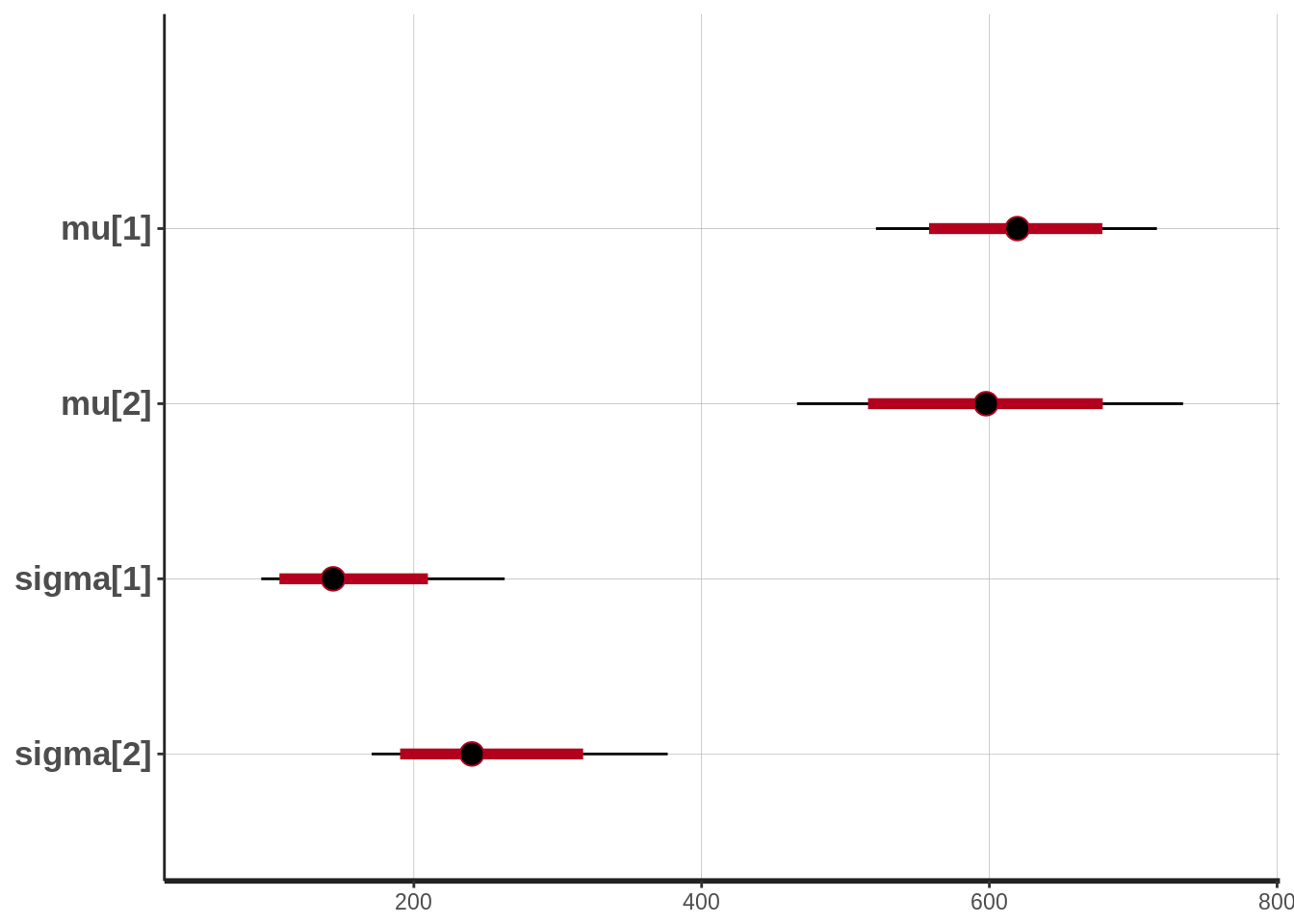This should give you the same result as in question 4.

## Question 8

Amazingly we don’t have to change the model at all from question 7, we can just rerun it with the new data. That is, if we were smart with how we defined the priors and instead of writing:

``````mu ~ uniform(0, 2000);
mu ~ uniform(0, 2000);``````

simply wrote `mu ~ uniform(0, 2000);`.

``````d <- data.frame(
milk = c(651, 679, 374, 601, 401, 609, 767, 709, 704, 679, 798, 1139, 529,
609, 553, 743, 151, 544, 488, 555, 257, 692, 678, 675, 538, 1061,
721, 595, 784, 877, 562, 800, 684, 741, 516),
group = c(1, 1, 1, 1, 1, 1, 1, 1, 1, 1, 2, 2, 2, 2, 2, 2, 2, 2, 2, 2, 2, 2,
2, 2, 2, 3, 3, 3, 3, 3, 3, 3, 3, 3, 3))

data_list <- list(y = d\$milk, x = d\$group, n = length(d\$milk),
n_groups = max(d\$group))

# Compiling and producing posterior samples from the model.
stan_samples <- stan(model_code = model_string, data = data_list)``````
``````# Plotting and summarizing the posterior distribution
stan_samples``````
``````## Inference for Stan model: 68927fed8cab7398aeed267d67b0a586.
## 4 chains, each with iter=2000; warmup=1000; thin=1;
## post-warmup draws per chain=1000, total post-warmup draws=4000.
##
##             mean se_mean    sd    2.5%     25%     50%     75%   97.5%
## mu     617.29    0.86 49.71  519.46  586.43  617.26  647.37  716.81
## mu     596.15    1.03 65.30  465.43  554.32  596.22  638.31  722.97
## mu     732.77    1.12 62.39  607.40  694.98  732.61  771.85  854.73
## sigma  152.15    0.90 45.75   92.82  121.33  143.76  171.13  264.76
## sigma  249.43    1.05 54.75  170.60  209.74  240.19  277.74  387.91
## sigma  189.41    1.15 57.09  113.59  150.74  177.56  214.53  337.04
## lp__     -184.81    0.05  1.94 -189.62 -185.80 -184.48 -183.37 -182.15
##          n_eff Rhat
## mu     3326    1
## mu     4000    1
## mu     3085    1
## sigma  2560    1
## sigma  2717    1
## sigma  2466    1
## lp__      1574    1
##
## Samples were drawn using NUTS(diag_e) at Sat Jan 14 23:37:35 2017.
## For each parameter, n_eff is a crude measure of effective sample size,
## and Rhat is the potential scale reduction factor on split chains (at
## convergence, Rhat=1).``````
``plot(stan_samples)``
``## ci_level: 0.8 (80% intervals)``
``## outer_level: 0.95 (95% intervals)``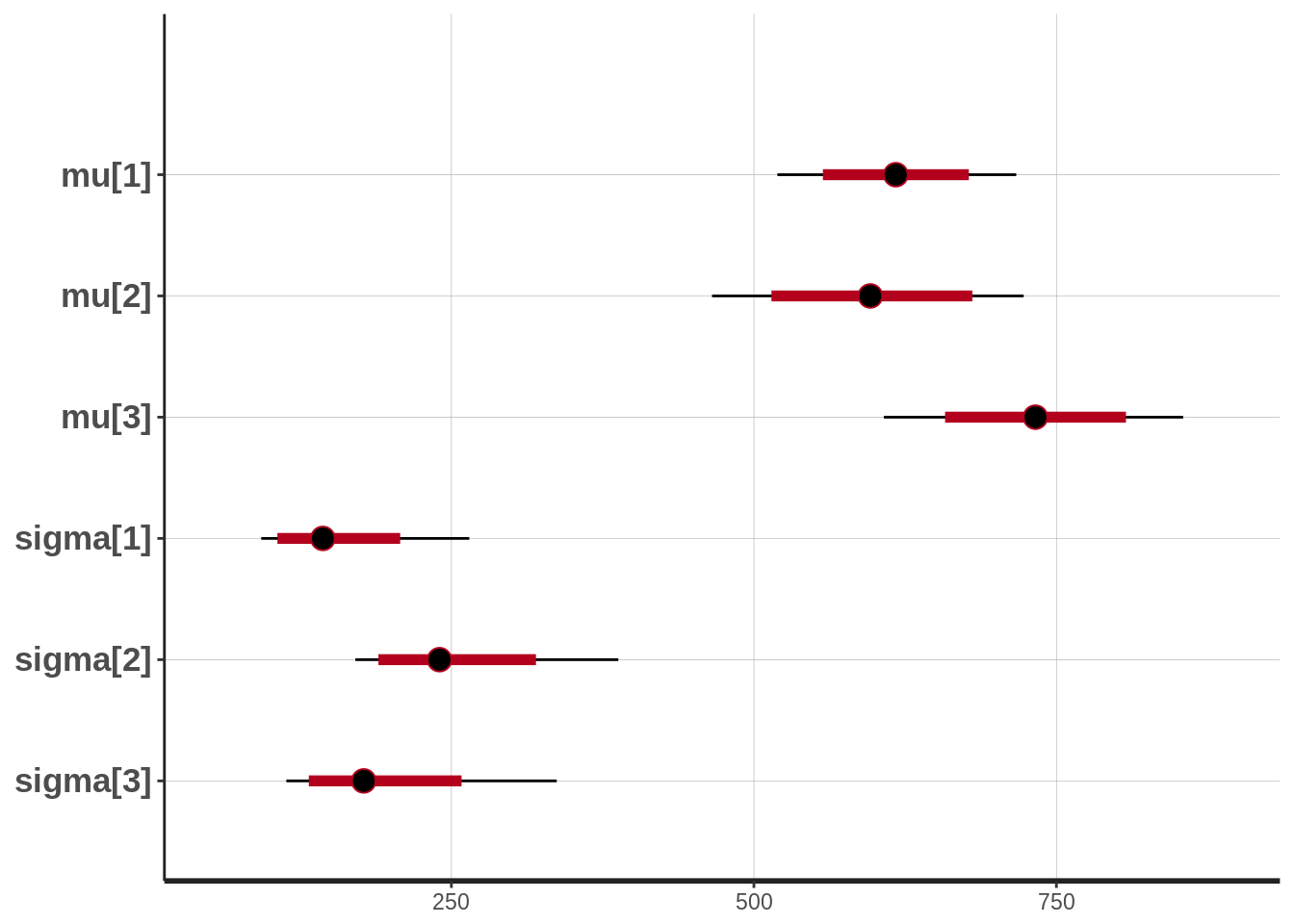``````# Now comparing the tree different groups.
s <- as.data.frame(stan_samples)
hist(s[,"mu"] - s[,"mu"] )``````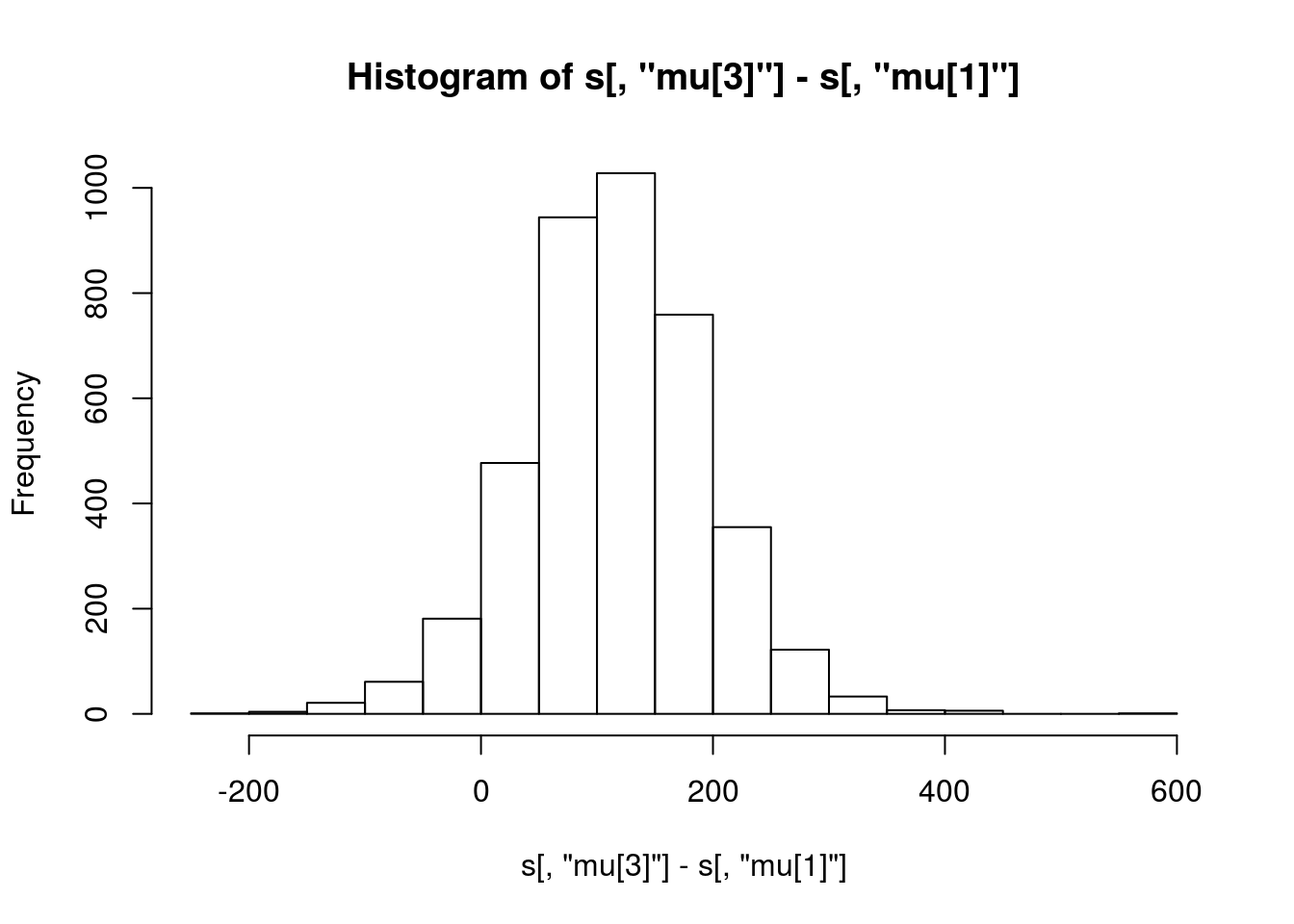``hist(s[,"mu"] - s[,"mu"] )``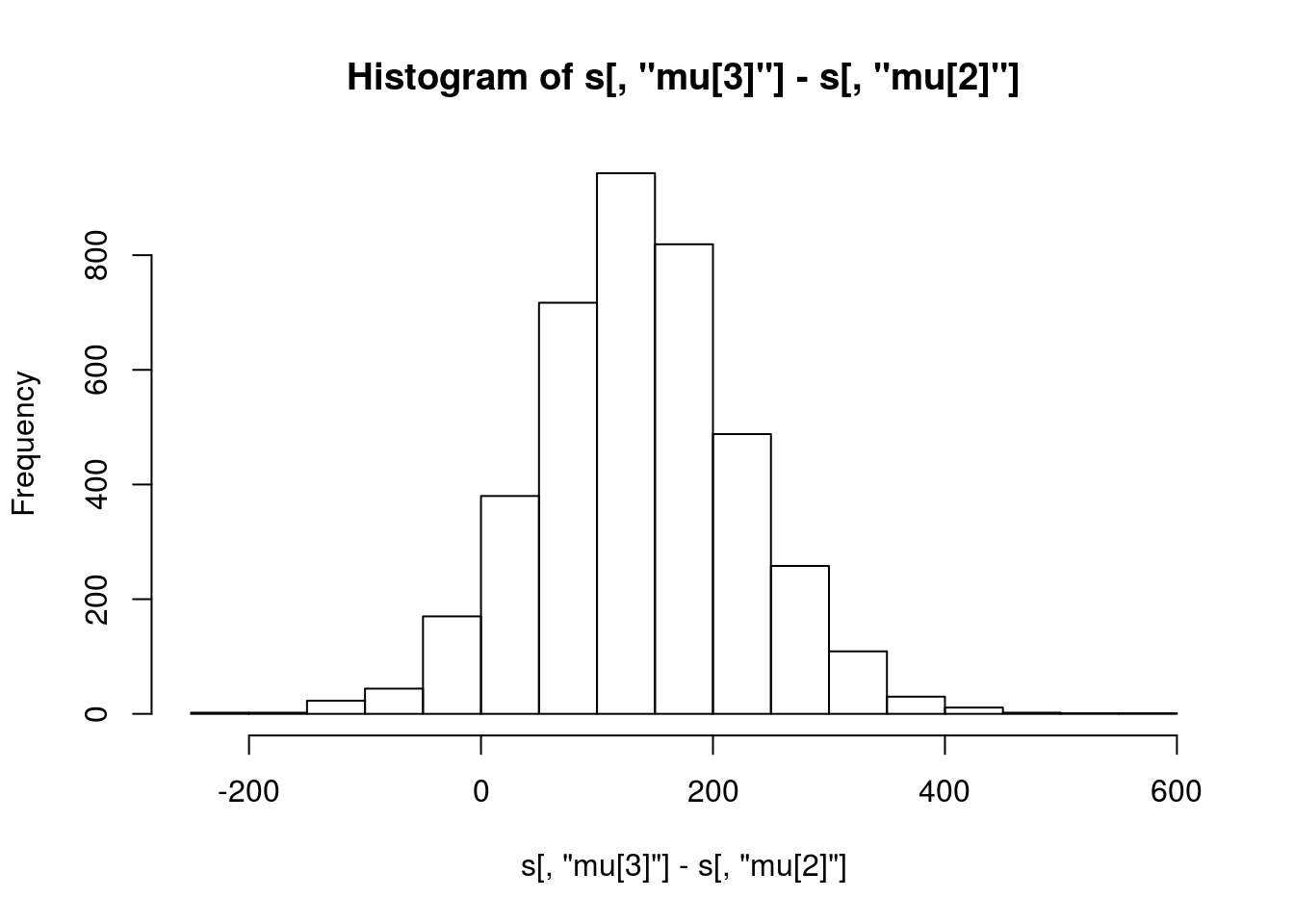``mean(s[,"mu"] - s[,"mu"] > 0)``
``##  0.933``
``mean(s[,"mu"] - s[,"mu"] > 0)``
``##  0.93975``

So it is pretty likely that diet 2 (`mu`) is better than both diet 1 (`mu`) and using no special diet (`mu`).

## Question 9

So, let’s change the model from question 7 into a regression model!

``````# The Stan model as a string.
model_string <- "
data {
int n;
vector[n] x;
vector[n] y;
}

parameters {
real beta0;
real beta1;
real<lower=0> sigma;
}

model {
vector[n] mu;
beta0 ~ uniform(-1000, 1000);
beta1 ~ uniform(-1000, 1000);
sigma ~ uniform(0, 1000);
mu = beta0 + beta1 * x;
y ~ normal(mu, sigma);
}

generated quantities {
}
"

d <- data.frame(milk = c(685, 691, 476, 1151, 879, 725, 1190, 1107, 809, 539,
298, 805, 820, 498, 1026, 1217, 1177, 684, 1061, 834),
hours = c(3, 7, 6, 10, 6, 5, 10, 11, 9, 3, 6, 6, 3, 5, 8, 11,
12, 9, 5, 5))
data_list <- list(y = d\$milk, x = d\$hours, n = length(d\$milk))

# Compiling and producing posterior samples from the model.
stan_samples <- stan(model_code = model_string, data = data_list)``````
``````# Plotting and summarizing the posterior distribution
stan_samples``````
``````## Inference for Stan model: 8ff03882e9f9a899c2e5b85844a34427.
## 4 chains, each with iter=2000; warmup=1000; thin=1;
## post-warmup draws per chain=1000, total post-warmup draws=4000.
##
##          mean se_mean     sd    2.5%     25%     50%     75%   97.5% n_eff
## beta0  402.33    3.97 139.10  128.52  312.96  399.96  490.73  674.56  1227
## beta1   61.59    0.52  18.36   24.78   49.98   61.89   73.39   97.31  1224
## sigma  223.08    1.00  40.10  161.71  195.05  217.39  242.89  320.51  1597
## lp__  -111.98    0.04   1.38 -115.66 -112.55 -111.62 -110.99 -110.47  1278
##       Rhat
## beta0    1
## beta1    1
## sigma    1
## lp__     1
##
## Samples were drawn using NUTS(diag_e) at Sat Jan 14 23:38:19 2017.
## For each parameter, n_eff is a crude measure of effective sample size,
## and Rhat is the potential scale reduction factor on split chains (at
## convergence, Rhat=1).``````
``plot(stan_samples)``
``## ci_level: 0.8 (80% intervals)``
``## outer_level: 0.95 (95% intervals)``